# Difference between revisions of "Tait equation of state"

The Tait equation is an equation of state. The equation was originally published by Peter Guthrie Tait in 1888. (Yuan-Hui Li, 15 May 1967, Equation of State of Water and Sea Water, Journal of Geophysical Research 72 (10), p. 2665.) It may be written as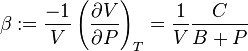$\beta := \frac{-1}{V} \left ( \frac{\partial V}{\partial P} \right )_T = \frac{1}{V} \frac{C}{B+P}$

or in the integrated form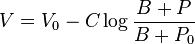$V = V_0 - C \log \frac{B+P}{B+P_0}$

where

•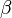$\beta$ is the compressibility.
•$V \$ is the specific volume.
•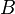$B \$ and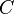$C \$ are functions of temperature that are independent of pressure.